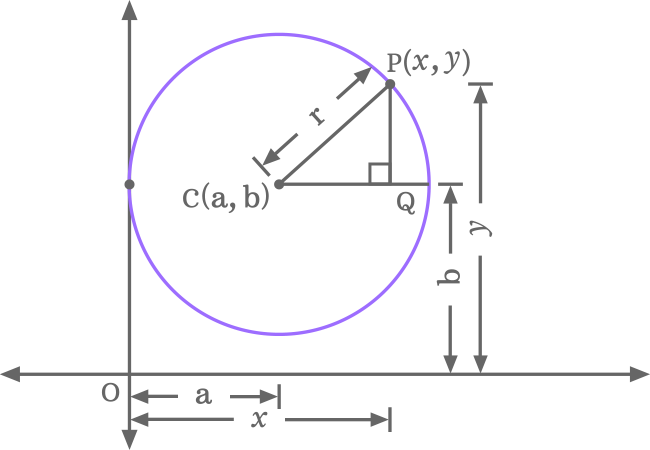# Proof for Equation of a Circle when the circle touches the y-axis

The equation of a circle when the circle touches the y-axis is written in one of the following two forms in mathematics.

$(1).\,\,\,$ $(x-a)^2+(y-b)^2 \,=\, a^2$

$(2).\,\,\,$ $(x-h)^2+(y-k)^2 \,=\, h^2$

Let us learn the geometric procedure to know how to prove the equation of a circle in mathematical form when the circle is touching the $y$-axis in two dimensional space.

### Construct a Triangle inside a Circle in a Quadrant

A right triangle (or right angled triangle) should has to construct geometrically inside a circle when the circle touches the $y$-axis at a particular point in a quadrant of the two dimensional Cartesian coordinate system for deriving the equation of circle.1. Draw a circle in such a way that it touches the vertical $y$-axis at a point in the first quadrant of two dimensional cartesian coordinate system.
2. Let $C$ be a point, which represents the center (or centre) of the circle. Suppose, the centre $C$ is $a$ and $b$ units away from origin in the horizontal and vertical directions. Hence, the center $C$ in coordinate form is written as $C(a, b)$ mathematically.
3. Let $P$ be a point on the circumference of the circle and the point $P$ is $x$ units away from the origin in horizontal direction, and is also $y$ units away from the origin in vertical direction. Therefore, the point $P$ in coordinate form is written as $P (x, y)$ in mathematics.
4. Join the point $P$ and centre $C$ by a straight line. The length of the line segment that joins the both points $C$ and $P$ is the radius of the circle, and it is taken as $r$ units. Draw a straight line horizontally from center $C$ and also draw a line vertically from point $P$ towards $x$-axis in such a way that they both got intersected at a point $Q$. Geometrically, a right triangle $(\Delta PCQ)$ is constructed.

### Evaluate the Length of each side of Triangle

In $\Delta PCQ$, $\overline{PQ}$, $\overline{CQ}$ and $\overline{CP}$ are the opposite side, adjacent side and hypotenuse, and the lengths of them are written as $PQ$, $CQ$ and $CP$ respectively in mathematics.Now, let us find the length of every side of the right angled triangle in algebraic form.

1. $CQ$ $\,=\,$ $OQ\,–\,OC$ $\,=\,$ $x \,–\,a$
2. $PQ$ $\,=\,$ $OP\,–\,OQ$ $\,=\,$ $y\,-\,b$
3. $CP \,=\, r$.

### Express the mathematical Relation between them

In this right triangle, the length of each side is known to us. So, the relation between three sides can be written in mathematical form by using the Pythagorean Theorem.${CP}^2$ $\,=\,$ ${CQ}^2+{PQ}^2$

Now, replace the length of each side in the above equation.

$r^2$ $\,=\,$ $(x \,-\, a)^2$ $+$ $(y \,-\, b)^2$

$\implies$ $(x-a)^2$ $+$ $(y-b)^2$ $\,=\,$ $r^2$

Here, $OC \,=\, r \,=\, a$.

$\,\,\,\therefore\,\,\,\,\,\,$ $(x-a)^2$ $+$ $(y-r)^2$ $\,=\,$ $r^2$

This equation can also be written as follows.

$\,\,\,\therefore\,\,\,\,\,\,$ $(x-a)^2$ $+$ $(y-b)^2$ $\,=\,$ $a^2$

The above two equations express the equation of a circle when the circle is touching the vertical $y$-axis at a point.

Use the square of difference formula to expand the square of difference of the terms.

$\implies$ $x^2$ $+$ $a^2$ $-$ $2ax$ $+$ $y^2$ $+$ $b^2$ $–$ $2by$ $\,=\,$ $a^2$

$\implies$ $x^2$ $+$ $y^2$ $-$ $2ax$ $–$ $2by$ $+$ $a^2$ $+$ $b^2$ $\,=\,$ $a^2$

$\implies$ $x^2$ $+$ $y^2$ $-$ $2ax$ $–$ $2by$ $+$ $b^2$ $+$ $a^2$ $\,=\,$ $a^2$

$\implies$ $x^2$ $+$ $y^2$ $-$ $2ax$ $–$ $2by$ $+$ $b^2$ $+$ $a^2$ $-$ $a^2$ $\,=\,$ $0$

$\implies$ $x^2$ $+$ $y^2$ $-$ $2ax$ $–$ $2by$ $+$ $b^2$ $+$ $\cancel{a^2}$ $-$ $\cancel{a^2}$ $\,=\,$ $0$

$\,\,\,\therefore\,\,\,\,\,\,$ $x^2$ $+$ $y^2$ $-$ $2ax$ $–$ $2by$ $+$ $b^2$ $\,=\,$ $0$

This equation represents the circle’s equation when the circle touches the $y$-axis at a point and it can also be written in the following form by replacing the $a$ with $r$.

$\,\,\,\therefore\,\,\,\,\,\,$ $x^2$ $+$ $y^2$ $-$ $2rx$ $–$ $2by$ $+$ $b^2$ $\,=\,$ $0$

#### Alternative form

The equation of a circle when the circle is touching the $y$-axis is also written in the following two forms if the centre (or center) is considered as $C (h, k)$.

### Simple form

$(x-h)^2+(y-k)^2 \,=\, h^2$

### Expansion

$x^2$ $+$ $y^2$ $-$ $2hx$ $–$ $2ky$ $+$ $k^2$ $\,=\,$ $0$

Latest Math Topics
Jun 26, 2023
Jun 23, 2023

Latest Math Problems
Jul 01, 2023
Jun 25, 2023
###### Math Questions

The math problems with solutions to learn how to solve a problem.

Learn solutions

Practice now

###### Math Videos

The math videos tutorials with visual graphics to learn every concept.

Watch now

###### Subscribe us

Get the latest math updates from the Math Doubts by subscribing us.